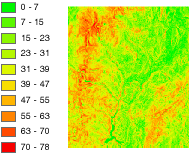# Slope function

## Overview

Slope represents the rate of change of elevation for each digital elevation model (DEM) cell. It's the first derivative of a DEM.## Notes

By default, the slope appears as a grayscale image. You can add the Colormap function to specify a particular color scheme, or allow the person viewing the mosaic to modify the symbology with their own color scheme.

This Slope function uses an accelerated ATan function. It is six times faster, and the approximation error is always less than 0.3 degrees.

## Parameters

The inputs for this function are the following:

Parameter nameDescription

DEM

The input elevation dataset.

Scaling

The inclination of slope can be output as either a value in degrees, or percent rise. There are three options for the Scaling:

• Degree—The inclination of slope is calculated in degrees. The values range from 0 to 90.

• PercentRise—The inclination of slope is calculated as percentage values. The values range from 0 to infinity. A flat surface is 0 percent rise, whereas a 45-degree surface is 100 percent rise. As the surface becomes more vertical, the percent rise becomes increasingly larger.

• Scaled—The inclination of slope is calculated the same as DEGREE, but the z-factor is adjusted for scale. It uses the Pixel Size Power and Pixel Size Factor values, which account for the resolution changes (scale) as the viewer zooms in and out. This is recommended when using worldwide datasets—particularly when using slope as a surface for visualization.

The z-factor is adjusted using the following equation:

``Adjusted Z Factor = (Z Factor) + (Pixel Size)Pixel Size Power × (Pixel Size Factor)``

Z Factor

The Z Factor is a scaling factor used to convert the elevation values for two purposes:

• To convert the elevation units (such as meters or feet) to the horizontal coordinate units of the dataset, which may be feet, meters, or degrees
• To add vertical exaggeration for visual effect

Disable default edge pixel interpolation

Using this option will avoid any resampling artifacts that may occur along the edges of a raster. The output pixels along the edge of a raster or beside pixels without a value will be populated with NoData; therefore, it is recommended that this option be used only when there are other rasters with overlapping pixels available. When overlapping pixels are available, these areas of NoData will display the overlapping pixel values instead of being blank.

• Unchecked—Bilinear resampling will be applied uniformly to resample your slope. This is the default.
• Checked—Bilinear resampling will be used within the slope, except along the edges of the rasters or beside pixels of NoData. These pixels will be populated with NoData, since it will reduce any sharp edge effects that may occur.

### Unit conversion

If the units of measure for the z (elevation) units are the same as the x,y (linear) units, the z-factor is 1.

If your data is in a projected coordinate system and your elevation and linear units of measure are different, you will need to define a z-factor to account for the difference.

To convert from feet to meters or vice versa, see the table below. For example, if your DEM's elevation units are feet and your mosaic dataset's units are meters, you would use a value of 0.3048 to convert your elevation units from feet to meters (1 foot = 0.3048 meters).

Conversion TypeConversion Factor

From feet to meters

0.3048

From meters to feet

3.28084

If your data is using a geographic coordinate system (such as a DTED in GCS_WGS 84), where the linear units are in degrees and your elevation is in meters, use a conversion factor of 1, and the system will automatically convert your linear degrees to meters. If your elevation units are not in meters, use the Arithmetic function to convert your elevation to meters before using the Slope function.

### Vertical exaggeration

To apply vertical exaggeration, you must multiply the conversion factor by the exaggeration factor. For example, if both elevation and dataset coordinates are meters and you want to exaggerate by a multiple of 10, the scaling factor would be the unit conversion factor (1.0) multiplied by the vertical exaggeration factor (10.0)—which would be a Z Factor of 10. If the elevation units are meters and the dataset is geographic (degrees), you would multiply the unit conversion factor (1.0) by the exaggeration factor (10.0)—which would be a Z Factor of 10.